Processing ......FreeComputerBooks.com Links to Free Computer, Mathematics, Technical Books all over the World

Statistical Mechanics of Lattice Systems: A Concrete Mathematical Introduction
Top Free C Programming Books 🌠 - 100% Free or Open Source!
• Title: Statistical Mechanics of Lattice Systems: A Concrete Mathematical Introduction
• Author(s) Sacha Friedli and Yvan Velenik
• Publisher: The MIT Press; 2nd edition (February 6, 2015); eBook (Updated Version, April 8 2022)
• Note: "We keep on this page an up-to-date list of corrections to the book"
• Hardcover: 640 pages
• eBook: PDF (590 pages) and PDF Files
• Language: English
• ISBN-10: 1107184827
• ISBN-13: 978-1107184824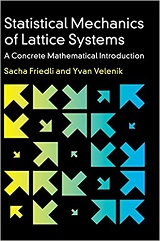Book Description

This motivating textbook gives a friendly, rigorous introduction to fundamental concepts in equilibrium statistical mechanics, covering a selection of specific models, including the Curie–Weiss and Ising models, the Gaussian free field, O(n) models, and models with Kać interactions. Using classical concepts such as Gibbs measures, pressure, free energy, and entropy, the book exposes the main features of the classical description of large systems in equilibrium, in particular the central problem of phase transitions.

It treats such important topics as the Peierls argument, the Dobrushin uniqueness, Mermin–Wagner and Lee–Yang theorems, and develops from scratch such workhorses as correlation inequalities, the cluster expansion, Pirogov–Sinai Theory, and reflection positivity.

Written as a self-contained course for advanced undergraduate or beginning graduate students, the detailed explanations, large collection of exercises (with solutions), and appendix of mathematical results and concepts also make it a handy reference for researchers in related areas.

• Sacha Friedli is Associate Professor of Mathematics at the Universidade Federal de Minas Gerais, Brazil. His current research interests are in statistical mechanics, mathematical physics, and Markov processes.
• Yvan Velenik is Professor of Mathematics at the Université de Genève. His current work focuses on applications of probability theory to the study of classical statistical mechanics, especially lattice random fields and random walks.
Reviews, Ratings, and Recommendations: Related Book Categories: Read and Download Links:Similar Books:
•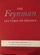The Feynman Lectures on Physics (Richard P. Feynman, et al.)

Ranging from the most basic principles of Newtonian physics through such formidable theories as general relativity and quantum mechanics, Feynman's lectures stand as a monument of clear exposition and deep insight.

•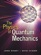The Physics of Quantum Mechanics (James Binney, et al.)

This book aims to give students a good understanding of how quantum mechanics describes the material world. It shows that the theory follows naturally from the use of probability amplitudes to derive probabilities.

•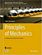Principles of Mechanics: Fundamental University Physics

This textbook takes the reader step-by-step through the concepts of mechanics in a clear and detailed manner. Many proofs and examples are included to help the reader grasp the fundamentals fully, paving the way to deal with more advanced topics.

•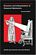Structure and Interpretation of Classical Mechanics

This innovative textbook concentrates on developing general methods for studying the behavior of classical systems. It focuses on the phenomenon of motion and makes extensive use of computer simulation in its explorations of the topic.

•Variational Principles in Classical Mechanics (Douglas Cline)

This book introduces variational principles and their application to classical mechanics. The relative merits of the intuitive Newtonian vectorial formulation, and the more powerful variational formulations are compared.

•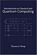Introduction to Classical and Quantum Computing (Tom Wong)

This book is for students who want to learn quantum computing beyond a conceptual level, but who lack advanced training in mathematics. The only prerequisite is trigonometry, and mathematics beyond that will be covered.

•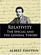Relativity: The Special and General Theory (Albert Einstein)

The great physicist himself disclaimed this exclusionary view, and in this book, he explains both theories in their simplest and most intelligible form for the layman not versed in the mathematical foundations of theoretical physics.

•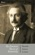The Einstein Theory of Relativity (Hendrik A. Lorentz)

The books published on the subject are so technical that only a person trained in pure physics and higher mathematics is able to fully understand them. In order to make a popular explanation of this far-reaching theory available, the present book is published.

•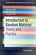Introduction to Random Matrices: Theory and Practice

This is a book for absolute beginners. The aim is to provide a truly accessible introductory account of Random Matrix theory. Most chapters are accompanied by Matlab codes to guide readers through the numerical check of most analytical results.

•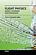Flight Physics - Models, Techniques and Technologies (K. Volkov)

Focuses on the synthesis of the fundamental disciplines and practical applications involved in the investigation, description, and analysis of aircraft flight including applied aerodynamics, aircraft propulsion, flight performance, stability, and control.

•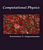Computational Physics and Scientific Computing: C++ or Fortran

This book is an introduction to the computational methods used in physics, but also in other scientific fields. Both C++ or Fortran are used for programming the core programs and data analysis is performed using the powerful tools of the Gnu/Linux environment.

•Computational Physics with Python (Eric Ayars)

This book provides an unusually broad survey of the topics of modern computational physics. Its philosophy is rooted in learning by doing, with new scientific materials as well as with the Python programming language.

Book Categories
 :All CategoriesTop Free BooksRecent BooksMiscellaneous BooksComputer EngineeringComputer LanguagesComputer ScienceData Science/DatabasesJava and Java EE (J2EE)Linux and UnixMathematicsMicrosoft and .NETMobile ComputingNetworking and CommunicationsSoftware EngineeringSpecial TopicsWeb Programming
Other Categories